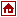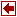Authors: Dash Associates Ltd Blisworth House Church Lane Blisworth Northants NN7 3BX, UK Phone: +44 1604 858993 Fax: +44 1604 858147 Email: info@dash.co.uk

Description

An XPRESS model is composed by sections (composed by sequence of XPRESS language statements) from:

• Bounds;
• Columns;
• Constraints;
• Data;
• DiskData;
• Indices;
• Let Section;
• Model Section;
• Rows;
• Set;
• Sets;
• Tables;
• Variables.

In the Bounds Sectionsimple upper and lower bounds and fixed and free values may be specified for LP and IP variables. The IP extension allows specification of integer, binary, partial integer and semi-continuous variables

The Columns Section The ROWS and COLUMNS sections are intended primarily for users who are accustomed to formulating their models columnwise (i.e. activity oriented). It is unlikely that other users will need to employ these sections.

The COLUMNS section declares coefficients of a decision variable for some or all of the constraints in which it occurs, i.e. it specifies the matrix elements by column. It may also be used to declare alternative vectors of Right-Hand-Side (RHS) coefficients.

In the Constraints Section are defined the constraints acting upon the decision variables of the model.

In the Data Section are initialised data tables which must have been defined previously in a TABLES section.

The DiskData Section is used to initialise data tables within the model from external data sources. The tables must have been defined previously in a TABLES section.

The Indices Section defines names and sizes of index sets.

In the Let Section are defined symbols which can be used later on in the model.

The Model Section identifies the model.

The Rows Section The ROWS and COLUMNS sections are intended primarily for users who are accustomed to formulating their models columnwise (i.e. activity oriented). It is unlikely that other users will need to employ these sections.

The Set Section is used to set or change the default options within mp-model.

The Sets Section defines Special Ordered Sets (SOS) of types 1 and 2 for variables in the model. There are two types available:

SOSs of Type 1. These are sets of variables of which at most one may be positive at an integer feasible solution. Typically these would correspond to mutually exclusive decisions, for example, choosing the month in which to start a major project. Associated with each set of variables there has to be some characteristic which orders the set members. If this is expressed as a (real) number, then it is specified by the coefficient of that variable in the reference row of the set to which it belongs.

SOSs of Type 2. These are sets of variables in which at most two can be positive at an integer feasible solution. Moreover, any positive variables must be 'adjacent' in a sense defined below. Their main use is in non-linear programming and we shall illustrate it by modelling a non-linear function of a single argument, f(x).

Tables Section defines data tables to be used in mp-model. Tables can have up to seven subscripts, can be sparse or dense and of single or double precision.

The Variables Section defines variables to be used in the model specification. Variables can have up to seven subscripts and can be defined to be sparse.

References

• Josef Kallrath and John M. Wilson, Business Optimisation Using Mathematical Programming, Macmillan 1997, ISBN 0-333-67623-8.

This book provides a practical and theoretical introduction to business optimisation using mathematical programming - linear programming, integer programming and other approaches. The focus of the book is primarily on models, model applications and individual case studies rather than algorithmic details. However, because the success of solution of complex problems requires efficient problem solving methods, models and algorithms are kept closely connected in the book. The book emphasises the importance of model formulation and makes extensive use of case studies which are either directly based on real applications or are simplified versions of realistic problems. Throughout the book problems are solved using the mathematical programming software XPRESS-MP and a full description of the use of this system is included. A CD-ROM containing a version of XPRESS-MP and models of solved problems is included with the book.

• H. P. Williams, Model Building in Mathematical Programming Third Edition Revised, Faculty of Mathematical Studies, University of Southampton, UK , Wiley, 1993, ISBN 0471 94111 5

This extensively revised and updated edition discusses the general principles of model building in mathematical programming and shows how they can be applied by using twenty simplified but practical problems from widely different contexts. Suggested formulations and solutions are given in the later part of the book, together with some computational experience to give the reader a feel for the computational difficulty of solving that particular type of model.

Aimed at undergraduates, postgraduates, research students and managers, this book illustrates the scope and limitations of mathematical programming, and shows how it can be applied to real situations. By emphasizing the importance of the building and interpreting of models rather than the solution process, the author attempts to fill a gap left by the many works which concentrate on the algorithmic side of the subject. The mathematical programming system MPSX has been replaced by XPRESS-MP in this revised edition.